# Problems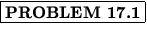An aluminum wire with a cross-sectional area of 4 x 10- 6 m 2 carries a current of 5 A. Find the drift speed of the electrons in the wire, assuming each aluminum atom contributes on electron. The density of aluminum is 2.7 g/cm 3.

Solution:
For this we will use

I = nqvdA.

To find the number of charges per unit volume, n , we find from the periodic table that the atomic mass of aluminum is 26.98 g/mol. Since 1 mol of a substance contains Avagadro's number NA = 6.02 x 1023 of particles, we find

n = 6.02 x 1023xx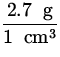x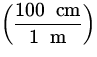3 = 6.02 x 1028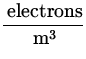We then find

vd =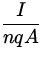=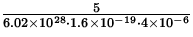= 1.3 x 10- 4 m / s .

Thus, the average drift speed is about 0.13 mm/s.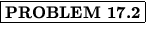Suppose one wants to make a 0.5resistor out of 1 g of Copper. If the resistor is a uniform cylinder, what is the diameter and length required?

Solution:
We will relate the resistance R to the resistivity by

R =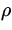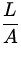,

where for Copper= 1.7 x 10- 8m . Since Copper has a density of 8.95 g/cm 3, we know that the volume V of Copper present is

V =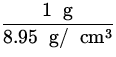x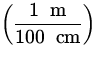3 = 1.12 x 10- 7 m 3.

Since V = LA , we then find

R ==L =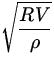= =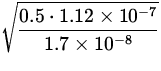= 1.8 m .

We can then also find the diameter d required as

A ==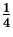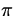d 2d =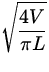== 2.8 x 10- 4 m .

Thus, the wire must have a length of 1.8 m and a diameter of 2.8 x 10- 4 m.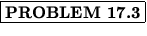A small motor draws a current of 2 A from a 120 V line. Assuming 100% efficiency, what is the cost of operating the motor for 10 hours if the power company charges $0.050/kWh? Solution: The motor has a power output of P = VI = 2 x 120 = 240 W . Operating it for 10 hours will consume an amount of energy E = Pt = 240 W x 10 h x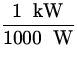= 2.4 kWh . It will thus cost 2.4 x$0.05=\$.12 to operate the motor.Find the current through R4 in the circuit in Fig. 17.9 if V = 30 V, R1 = 12, R2 = 18, R3 = 9, and R4 = 6.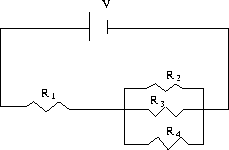Solution:
We first reduce the four resistors to one equivalent resistance. The resistors R1 , R2 , and R3 are in parallel; they have an equivalent resistance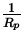=+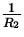+=++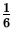Rp = 3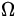.

This equivalent resistance is then in series with R1 ; the equivalent resistance of these two resistors in series is

R = R1 + Rp = 12 + 3 = 15.

Thus, the equivalent resistance of the four resistors is 15. The current flowing through this equivalent circuit is thus

I ==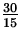= 2 A ,

from which we deduce the potential difference across the equivalent resistance Rp = 3as

Vp = IRp = 2 x 3 = 6 V .

This potential difference is the same across R2 , R3 , and R4 , and in particular we have through R4

I4R4 = 6I4 =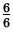= 1 A .

Thus, the current through R4 is 1 A.Find the power lost in the 50resistor in the circuit in Fig. 17.10.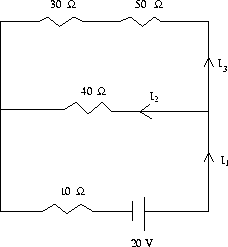Solution:
We begin by labelling the unknown currents as indicated. Applying Kirchhoff's junction rule, we find

I1 = I2 + I3.

We next use Kirchhoff's loop rule; for the top loop, going clockwise, we find

30I3 + 50I3 - 40I2 = 0I3 =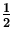I2,

while for the bottom loop, also going clockwise,

40I2 - 20 + 10I1 = 0I1 = 2 - 4I2.

Substituting these last two relations into the first yields

2 - 4I2 = I2 +I2I2 =A ,

from which follows
 I3 =I2 =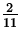A , I1 = 2 - 4I2 =A .
The fact that all three currents came out positive indicates the directions we assumed for them were correct.

As a check on our algebra, we can verify that by going around the outer loop

30I3 + 50I3 - 20 + 10I1 = 0,

as it should. We then know the current through the 50resistor to be I3 =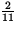A, from which we find the power lost as

P = I 2R =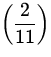2 x 50 = 1.65 W .

Thus, the 50resistor dissipates 1.65 W of power.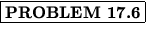Find the currents through all three resistors in the circuit in Fig. 17.11.Solution:
We begin by labeling the unknown currents as indicated. Applying Kirchhoff's junction rule, we find

I1 + I3 = I2.

We next use Kirchhoff's loop rule; for the top loop, going clockwise, we find

20 - 100I2 - 20I3 = 0I3 = 1 - 5I2,

while for the bottom loop, also going clockwise,

100I2 - 60 + 10I1 = 0I1 = 6 - 10I2.

Substituting these last two relations into the first yields

(1 - 5I2) + (6 - 10I2) = I2I2 =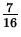A ,

from which follows
 I3 = 1 - 5I2 = -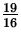A , I1 = 6 - 10I2 =A .
In this case the value obtained for I3 is negative, which indicates that our initial guess for the direction of I3 was wrong. We also see by this that the 20 V battery is in fact being charged.

As a check on our algebra, we can verify that by going around the outer loop

- 20I3 + 20 - 60 + 10I1 = 0,

as it should. Note that for this check we must explicitly use the negative value found for I3 .